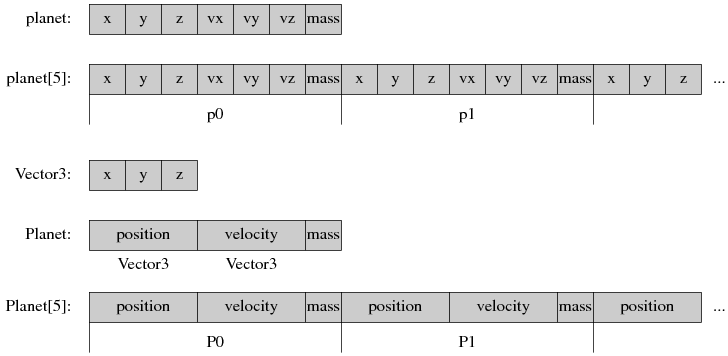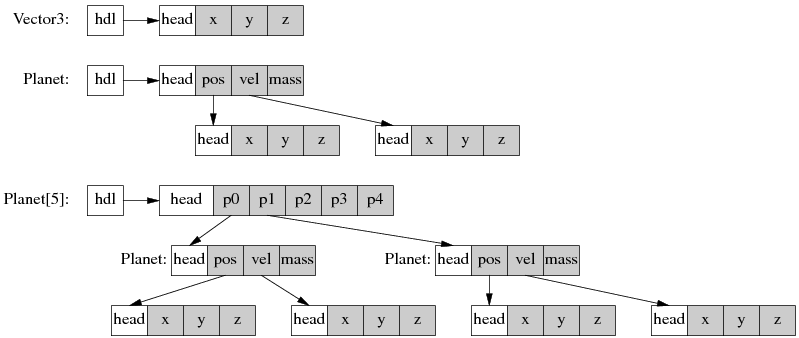# 陈硕的 Blog

## C++ 工程实践(9)：数据抽象

http://blog.csdn.net/Solstice  http://weibo.com/giantchen

1. 什么是数据抽象？它与面向对象有何区别？
2. 数据抽象所需的语言设施
3. 数据抽象的例子

# 什么是数据抽象

“支持数据抽象”一直是C++语言的设计目标，Bjarne Stroustrup 在他的《The C++ Programming Language》第二版（1991年出版）中写道[2nd]：

The C++ programming language is designed to

• be a better C
• support data abstraction
• support object-oriented programming

C++ is a general-purpose programming language with a bias towards systems programming that

• is a better C,
• supports data abstraction,
• supports object-oriented programming, and
• supports generic programming.

http://www.softwarepreservation.org/projects/c_plus_plus/index.html#cfront 可以找到 C++ 的早期文献，其中有一篇 Bjarne Stroustrup 在 1984 年写的 《Data Abstraction in C++》 http://www.softwarepreservation.org/projects/c_plus_plus/cfront/release_e/doc/DataAbstraction.pdf 。在这个页面还能找到 Bjarne 写的关于 C++ 操作符重载和复数运算的文章，作为数据抽象的详解与范例。可见 C++ 早期是以数据抽象为卖点的，支持数据抽象是C++相对于C的一大优势。

## C++ 标准库中的数据抽象

C++ 标准库里  complex<> 、pair<>、vector<>、list<>、map<>、set<>、string、stack、queue 都是数据抽象的例子。vector 是动态数组，它的主要操作有 push_back()、size()、begin()、end() 等等，这些操作不仅含义清晰，而且计算复杂度都是常数。类似的，list 是链表，map 是有序关联数组，set 是有序集合、stack 是 FILO 栈、queue是 FIFO 队列。“动态数组”、“链表”、“有序集合”、“关联数组”、“栈”、“队列”都是定义明确（操作、复杂度）的抽象数据类型。

## 数据抽象与面向对象的区别

ADT class 可以作为 Object-based/object-oriented class 的成员，但反过来不成立，因为这样一来 ADS class 的拷贝就失去意义了。

# 数据抽象所需的语言设施

## 全局函数与重载

C 语言可以定义全局函数，但是不能与已有的函数重名，也就没有重载。Java 没有全局函数，而且 Math class 是封闭的，并不能往其中添加 sin(Complex)。

## 拷贝控制(copy control)

copy control 是拷贝 stack a; stack b = a; 和赋值 stack b; b = a; 的合称。

C 语言没有 copy control，也没有办法防止拷贝，一切要靠程序员自己小心在意。FILE* 可以随意拷贝，但是只要关闭其中一个 copy，其他 copies 也都失效了，跟空悬指针一般。整个 C 语言对待资源（malloc 得到的内存，open() 打开的文件，socket() 打开的连接）都是这样，用整数或指针来代表（即“句柄”）。而整数和指针类型的“句柄”是可以随意拷贝的，很容易就造成重复释放、遗漏释放、使用已经释放的资源等等常见错误。这方面 C++ 是一个显著的进步，boost::noncopyable 是 boost 里最值得推广的库。

## 操作符重载

    public static BigInteger mean(BigInteger x, BigInteger y) {
BigInteger two = BigInteger.valueOf(2);
}

public static long mean(long x, long y) {
return (x + y) / 2;
}

## 效率无损

“抽象”不代表低效。在 C++ 中，提高抽象的层次并不会降低效率。不然的话，人们宁可在低层次上编程，而不愿使用更便利的抽象，数据抽象也就失去了市场。后面我们将看到一个具体的例子。

# 数据抽象的例子

C 语言版，完整代码见 https://gist.github.com/1158889#file_nbody.c，下面是代码骨干。planet 保存与行星位置、速度、质量，位置和速度各有三个分量，程序模拟几大行星在三维空间中受引力支配的运动。

struct planet
{
double x, y, z;
double vx, vy, vz;
double mass;
};

void advance(int nbodies, struct planet *bodies, double dt)
{
for (int i = 0; i < nbodies; i++)
{
struct planet *p1 = &(bodies[i]);
for (int j = i + 1; j < nbodies; j++)
{
struct planet *p2 = &(bodies[j]);
double dx = p1->x - p2->x;
double dy = p1->y - p2->y;
double dz = p1->z - p2->z;
double distance_squared = dx * dx + dy * dy + dz * dz;
double distance = sqrt(distance_squared);
double mag = dt / (distance * distance_squared);
p1->vx -= dx * p2->mass * mag;
p1->vy -= dy * p2->mass * mag;
p1->vz -= dz * p2->mass * mag;
p2->vx += dx * p1->mass * mag;
p2->vy += dy * p1->mass * mag;
p2->vz += dz * p1->mass * mag;
}
}
for (int i = 0; i < nbodies; i++)
{
struct planet * p = &(bodies[i]);
p->x += dt * p->vx;
p->y += dt * p->vy;
p->z += dt * p->vz;
}
}


C++ 数据抽象版，完整代码见 https://gist.github.com/1158889#file_nbody.cc，下面是代码骨架。

struct Vector3
{
Vector3(double x, double y, double z)
: x(x), y(y), z(z)
{
}

double x;
double y;
double z;
};

struct Planet
{
Planet(const Vector3& position, const Vector3& velocity, double mass)
: position(position), velocity(velocity), mass(mass)
{
}

Vector3 position;
Vector3 velocity;
const double mass;
};


void advance(int nbodies, Planet* bodies, double delta_time)
{
for (Planet* p1 = bodies; p1 != bodies + nbodies; ++p1)
{
for (Planet* p2 = p1 + 1; p2 != bodies + nbodies; ++p2)
{
Vector3 difference = p1->position - p2->position;
double distance_squared = magnitude_squared(difference);
double distance = std::sqrt(distance_squared);
double magnitude = delta_time / (distance * distance_squared);
p1->velocity -= difference * p2->mass * magnitude;
p2->velocity += difference * p1->mass * magnitude;
}
}
for (Planet* p = bodies; p != bodies + nbodies; ++p)
{
p->position += delta_time * p->velocity;
}
}


C struct 的成员是连续存储的，struct 数组也是连续的。C++ 尽管定义了了 Vector3 这个抽象，它的内存布局并没有改变，Planet 的布局和 C planet 一模一样，Planet[] 的布局也和 C 数组一样。# 更多的例子

• Date 与 Timestamp，这两个 class 的“数据”都是整数，各定义了一套操作，用于表达日期与时间这两个概念。
• BigInteger，它本身就是一个“数”。如果用 C++ 实现 BigInteger，那么阶乘函数写出来十分自然，下面第二个函数是 Java 语言的版本。
BigInteger factorial(int n)
{
BigInteger result(1);
for (int i = 1; i <= n; ++i) {
result *= i;
}
return result;
}

public static BigInteger factorial(int n) {
BigInteger result = BigInteger.ONE;
for (int i = 1; i <= n; ++i) {
result = result.multiply(BigInteger.valueOf(i));
}
return result;
}

• 图形学中的三维齐次坐标 Vector4 和对应的 4x4 变换矩阵 Matrix4，例如 http://www.ogre3d.org/docs/api/html/classOgre_1_1Matrix4.html
• 金融领域中经常成对出现的“买入价/卖出价”，可以封装为 BidOffer struct，这个 struct 的成员可以有 mid() “中间价”，spread() “买卖差价”，加减操作符，等等。

# 小结

posted on 2011-08-22 00:20  陈硕  阅读(5845)  评论(6编辑  收藏  举报